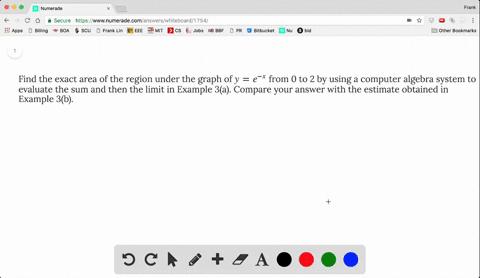### Find the exact area of the region under the graph…

01:32
FL
Problem 29

(a) Express the area under the curve $y = x^5$ from 0 to 2 as a limit.

(b) Use a computer algebra system to find the sum in your expression from part (a).

(c) Evaluate the limit in part (a).

(A). $\lim _{n \rightarrow \infty} \frac{64}{n^{6}} \sum_{i=1}^{n} i^{5}$
(B). $\frac{n^{2}(n+1)^{2}\left(2 n^{2}+2 n-1\right)}{12}$
(C). $\frac{32}{3}$

## Discussion

You must be signed in to discuss.

## Video Transcript

express this area's limit the leaves a computer part to you and Poppy and party. You can use computer to do it. I'Ll set up the formula for you. So here the general formula Sorry, my effects into grow from NATO P f X t x ISS limit on Goes to infinity It's rectangle has this lens on Were you survived and point Matthew So if a plus i b minus a over. So here we just plugging the exact number and functional We have bees to a zero half off a Ciro's or we'LL have this piece to a serious always tio I over on and here half off, half of access Excellent fifth power So hear this partial bit is to the fifth power.